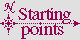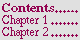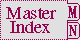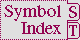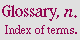#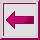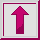Function LDIFF, TAILP

Syntax:

ldiff list object => result-list

tailp object list => generalized-boolean

Arguments and Values:

list---a list, which might be a dotted list.

object---an object.

result-list---a list.

generalized-boolean---a generalized boolean.

Description:

If object is the same as some tail of list, tailp returns true; otherwise, it returns false.

If object is the same as some tail of list, ldiff returns a fresh list of the elements of list that precede object in the list structure of list; otherwise, it returns a copy of list.

Examples:

``` (let ((lists '#((a b c) (a b c . d))))
(dotimes (i (length lists)) ()
(let ((list (aref lists i)))
(format t "~2&list=~S ~21T(tailp object list)~
~44T(ldiff list object)~%" list)
(let ((objects (vector list (cddr list) (copy-list (cddr list))
'(f g h) '() 'd 'x)))
(dotimes (j (length objects)) ()
(let ((object (aref objects j)))
(format t "~& object=~S ~21T~S ~44T~S"
object (tailp object list) (ldiff list object))))))))
>>
>>  list=(A B C)         (tailp object list)    (ldiff list object)
>>   object=(A B C)      T                      NIL
>>   object=(C)          T                      (A B)
>>   object=(C)          NIL                    (A B C)
>>   object=(F G H)      NIL                    (A B C)
>>   object=NIL          T                      (A B C)
>>   object=D            NIL                    (A B C)
>>   object=X            NIL                    (A B C)
>>
>>  list=(A B C . D)     (tailp object list)    (ldiff list object)
>>   object=(A B C . D)  T                      NIL
>>   object=(C . D)      T                      (A B)
>>   object=(C . D)      NIL                    (A B C . D)
>>   object=(F G H)      NIL                    (A B C . D)
>>   object=NIL          NIL                    (A B C . D)
>>   object=D            T                      (A B C)
>>   object=X            NIL                    (A B C . D)
=>  NIL
```

Side Effects:

Neither ldiff nor tailp modifies either of its arguments.

Affected By: None.

Exceptional Situations:

Should be prepared to signal an error of type type-error if list is not a proper list or a dotted list.

Notes:

If the list is a circular list, tailp will reliably yield a value only if the given object is in fact a tail of list. Otherwise, the consequences are unspecified: a given implementation which detects the circularity must return false, but since an implementation is not obliged to detect such a situation, tailp might just loop indefinitely without returning in that case.

tailp could be defined as follows:

``` (defun tailp (object list)
(do ((list list (cdr list)))
((atom list) (eql list object))
(if (eql object list)
(return t))))
```

and ldiff could be defined by:

```(defun ldiff (list object)
(do ((list list (cdr list))
(r '() (cons (car list) r)))
((atom list)
(if (eql list object) (nreverse r) (nreconc r list)))
(when (eql object list)
(return (nreverse r)))))
```

The following X3J13 cleanup issues, not part of the specification, apply to this section: